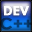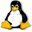Home Latest News Archive Tutorials C++ 2 3 4 Win32 Java OpenGL GLUT OpenGL ES 2 3 4 DirectX VB.NET HTML Photoshop 3dsmax Forums Projects Modelling Links About Us Search Recent Tutorials C / C++ Win32 OpenGL OpenGL ES GLUT## While Loop

Tutorials > C++ > While Loop

### Introduction

Many programs perform the same task a number of times. If you would like to run a section of code more than once, you could copy and paste the code the number of times that you want the code to be run. This limits you to only being able to run the code segment a specific number of times. This may also result in problems when you want to change the code in the code segment. We can use a feature known as loops to solve all of these issues.

There are different forms of loops that may be utilized. These will be discussed in the next few tutorials. For this tutorial, we will be discussing the while loop.

The while loop will continue to run while a particular statement evaluates to true.

The while loop takes the form :

while ( logical expression ) { statements }

Contents of main.cpp :

```#include <iostream>
#include <stdlib.h>
#include <string>

using namespace std;
```

There is nothing exciting about the code below. It just initializes some variables that we will use and allows the user to enter a number.

```int main()
{
int num = 0;
int count = 0;
string data = "";

cout << "Please enter a number : ";
cin >> num;
```

The next segment of code will cause the line starting with cout to loop until the count variable is greater than the number that was entered by the user.

The count variable is increased before it is printed out. As count is initialized to 0, the first value printed will therefore be 1. You should therefore see a list of numbers counting from 1 to the number that was entered.

If a number less than 1 is entered, nothing is displayed as count is already greater than the number that was entered.

```	while (count < num)
cout << ++count << endl;
```

The last piece of code is another example of how user input can determine how often a segment of code should be run. As data was initialized to "", it will enter the loop and will continue to ask the user for input until they type in q and press enter.

```	cout << endl;

while(data != "q")
{
cout << "Enter data (q to quit) : ";
cin >> data;
}

system("pause");

return 0;
}```

You should now be able to use the while loop to repeat segments of code. Other types of loops will be discussed in the next few tutorials.

Please let me know of any comments you may have : Contact Me

 Source Files :< Tutorial 14 - Switch Statement Tutorial 16 - Do-While Loop >